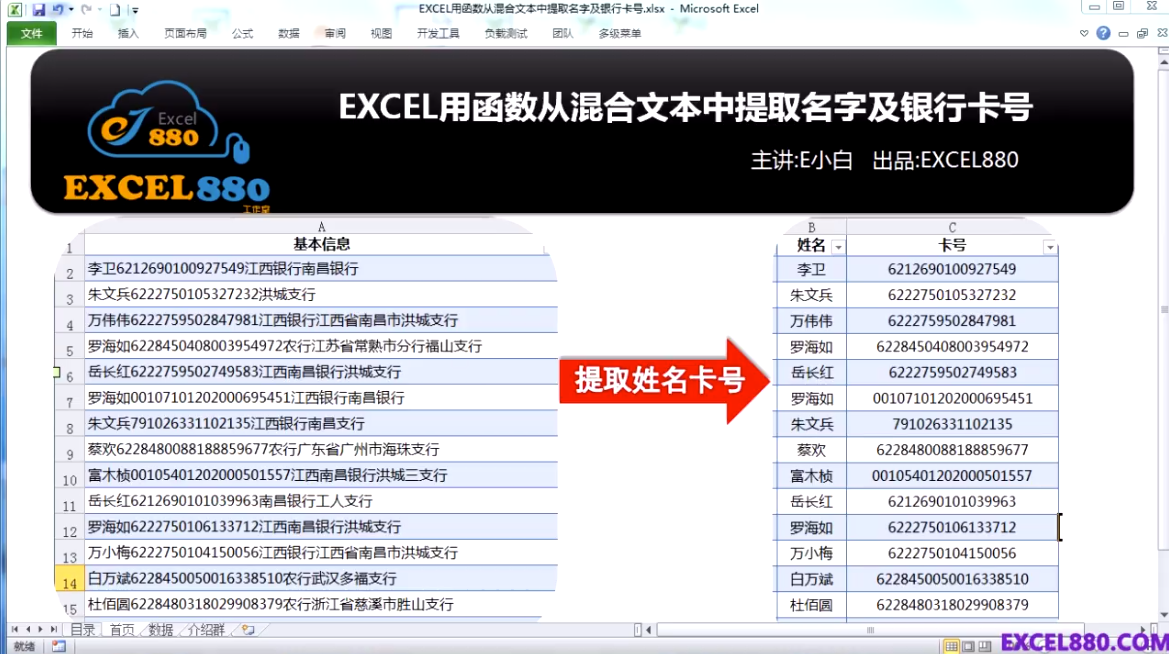# 【视频教程】EXCEL用函数从混合文本中提取名字及银行卡号 分列显示

2017年2月22日19:07:11 2 11,154 views

`=searchb("?",a2)`

`=2*len(a2)-lenb(a2)`

`= lenb(a2)-len(a2)`

=left(a2,searchb("?",a2)-1)  从左取 第一个数字位置减1个字符

=mid(a2,searchb("?",a2),2*len(a2)-lenb(a2)) 用的正是上面的套路